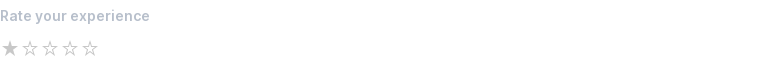# Rating¶

Use a rating component to capture a star-rating.

## Basic¶

Set `mode='rating'` to accept a star-rating.

By default, five stars are displayed.

``````stars = view(box('Rate your experience', mode='rating'))
``````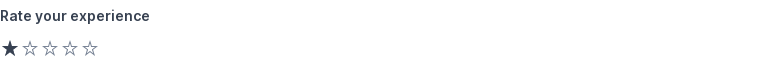## Set initial rating¶

Set `value=` to specify a default value.

``````stars = view(box('Rate your experience', mode='rating', value=3))
``````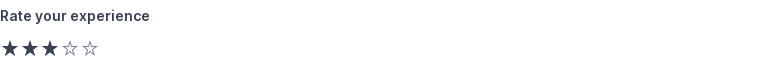## Allow zero stars¶

Set `min=0` to allow zero stars.

``````stars = view(box('Rate your experience', mode='rating', min=0))
``````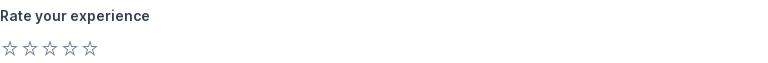## Set maximum number of stars¶

Set `max=` to increase the number of stars displayed.

``````stars = view(box('Rate your experience', mode='rating', value=3, max=10))
``````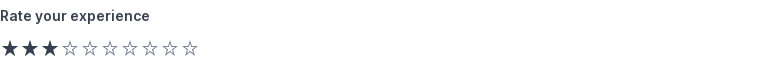## Combine min and max stars¶

`min=` and `max=` can be combined.

``````stars = view(box('Rate your experience', mode='rating', value=3, min=0, max=10))
``````## Set range¶

Set `range=` to a `(min, max)` tuple to control min/max stars.

This is a shorthand notation for setting `min=` and `max=` individually.

``````stars = view(box('Rate your experience', mode='rating', value=3, range=(0, 10)))
``````## Handle changes immediately¶

Add `live` to `mode` to handle changes immediately.

``````stars = 3
while True:
stars = view(
box('Rate your experience', mode='live rating', value=stars),Set `disabled=True` to disable.
``````view(box('Rate your experience', mode='rating', disabled=True))